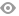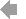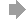﻿ Page 7 - MATINF Nr. 6
Basic HTML VersionView Full Version
Page 7 - MATINF Nr. 6
P. 7

``````Two Identities and their Consequences                                                           7

Proof. For a triangle, if in (1) we assume c = 0, then we have the well-known formulae   1

α    (s − a)(s − d)              α    s(s − b)
sin 2  =                  and    cos 2  =          .                   (4)

Making use of the double-angle identity for sine we have

Ê          Ê
p
s(s − b)   (s − a)(s − d)       s(s − a)(s − b)(s − d)
sin α = 2                             = 2                         .

Since ∆ 0 =  ad sin α , it follows
2
È
∆ 0 =   s(s − a)(s − b)(s − d).
As mentioned, the following proof of Brahmagupta’s formula is known. However, no further
generalizations of (1) are given in .

Theorem 3 (Brahmagupta). Given an cyclic quadrilateral, ABCD, with sides a, b, c, d and
semiperimeter, s, then its area is given by the formula

È
∆ 1 =    (s − a)(s − b)(s − c)(s − d).
Proof. Let α and γ are two opposite angles. The area of ABCD can be expressed as the sum of
the area of 4ABD and 4BCD, which in turn can be written as           ad sin α  +  bc sin γ  . Keeping in
2       2
mind that α and γ are supplementary and applying the formulae in (1) we have

◦
ad sin α   bc sin (180 − α)
∆ 1 =          +
2              2
ad sin α   bc sin α
=          +
2         2
α     α
= sin   cos (ad + bc)
2     2
Ê                Ê
(s − a)(s − d)   (s − b)(s − c)
È
=    (s − a)(s − b)(s − c)(s − d).

A bicentric quadrilateral is a convex quadrilateral that has both an incircle and a circumcircle
(see Figure 2). One characterization states that a convex quadrilateral ABCD with sides a, b,
c, d is bicentric if and only if opposite sides satisfy a + c = b + d and its opposite angles are
supplementary . Another property of a bicentric quadrilateral is that its area is given by
√
the formula    abcd. Six derivations of this formula can be found in [12, 13]. One derivation is
to use a + c = b + d in Brahmagupta’s Formula. Here we shall give a seventh proof which is
independent from Brahmagupta’s Formula.

Theorem 4. Given an bicentric quadrilateral, ABCD, with sides a, b, c and d, then its area is
given by the formula                            √
∆ 2 =   abcd.
1
For more implications in a triangle see .``````2   3   4   5   6   7   8   9   10   11   12